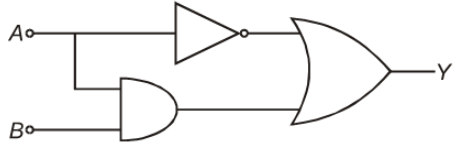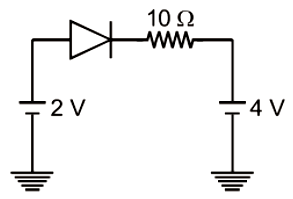Use of transistor as an amplifier works in the region -

1.  Cut off region

2.  Saturation region

3.  Passive region

4.  Active region

Concept Questions :-

Applications of Transistor
High Yielding Test Series + Question Bank - NEET 2020

Difficulty Level:

Which of the following is correct for n-type semiconductor?

1.  Electron is majority carriers and trivalent atoms are dopants.

2.  Electrons are majority carriers and pentavalent atoms are dopants.

3.  Holes are majority carriers and pentavalent atoms are dopants.

4.  Holes are majority carriers and trivalent atoms are dopants.

Concept Questions :-

Applications of PN junction
High Yielding Test Series + Question Bank - NEET 2020

Difficulty Level:

1.

2.

3.

4.  All of these

Concept Questions :-

Transistor
High Yielding Test Series + Question Bank - NEET 2020

Difficulty Level:

For a transistor amplifier, the power gain and voltage gain are 150 and 10 respectively. The current gain is-

1.

2.  $\frac{1}{15}$

3.  15

4.  1500

Concept Questions :-

Applications of Transistor
High Yielding Test Series + Question Bank - NEET 2020

Difficulty Level:

In semiconductors, which of the following gives the law of mass action (where symbols have their usual meanings)-

1.

2.

3.

4.

Concept Questions :-

Types of semiconductors
High Yielding Test Series + Question Bank - NEET 2020

Difficulty Level:

The conductivity of an n-type semiconductor whose density of conduction electrons is ${\mathrm{n}}_{\mathrm{e}}$, Density of holes is ${\mathrm{n}}_{\mathrm{h}}$, Mobility of conduction electrons is m and Mobility of holes is ${\mathrm{m}}_{\mathrm{h}},$ will be

1.

2.

3.

4.

Concept Questions :-

Types of semiconductors
High Yielding Test Series + Question Bank - NEET 2020

Difficulty Level:

When the temperature of the silicon sample is increased from  to , the conductivity of silicon will be

1.  Increased

2.  Decreased

3.  Remain same

4.  Zero

Concept Questions :-

Types of semiconductors
High Yielding Test Series + Question Bank - NEET 2020

Difficulty Level:

The output Y of the logic gate circuit shown in figure is best represented as(1) $\overline{)A}+\left(A.B\right)$

(2) $A+\overline{)\left(A+B\right)}$

(3) A+(A.B)

(4) $\overline{)A}+\overline{)\left(A.B\right)}$

Concept Questions :-

Logic gates
High Yielding Test Series + Question Bank - NEET 2020

Difficulty Level:

A transistor is used in common emitter mode in an amplifier circuit. When a signal of 10 mV is added to the base-emitter voltage, the base current changes by 15 $\mu$A and the collector current changes by 1.5 mA. The current gain b will be

1.  50

2.  48

3.  100

4.  200

Concept Questions :-

Applications of Transistor
High Yielding Test Series + Question Bank - NEET 2020

Difficulty Level:

The current through an ideal p-n junction diode shown in the circuit will be -1.  5 A

2.  0.2 A

3.  0.6 A

4.  Zero

Concept Questions :-

Applications of PN junction